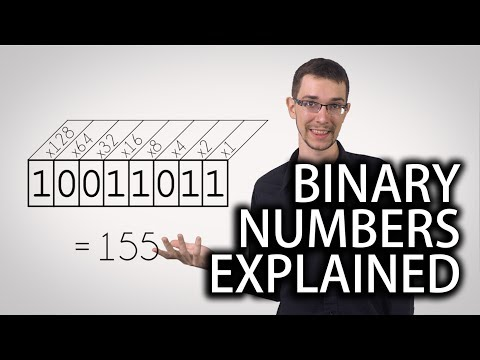## Binary Number System

Subject: Compulsory Maths

#### Overview

Binary number system consists of two digits 0 and 1 and its base is 2. Each digit or bit in binary number system can be 0 or 1. It also provides the information about the Conversion of binary numbers to decimal number, Conversion of decimal number to binary number and Alternative method fo binary number system.

#### Binary number system

Binary number system consists of two digits 0 and 1 and its base is 2. Each digit or bit in binary number system can be 0 or 1. Digital computer represents all kinds of data and information in the binary system.

#### Conversion of binary numbers to decimal number

1100112 = 1× 25 + 1× 24 + 0× 23 + 0× 22 + 1× 21 + 1× 20In order to convert a binary number into a decimal, it is expanded in the power of 2. Then by simplifyingthe expanded form of the binary number, we obtain a decimal number. For example,
10112 = 1× 23 + 0× 22 + 1× 21 + 1× 20
= 8 + 0 + 2 + 1
∴ 10112 = 11= 32 + 16 + 0 + 0 + 2 + 1
∴ 1100112 = 51

#### Conversion of decimal number to binary number

We can convert decimal number into a binary number by using the place value table of the binary system. For example:
Convert 30 into binary system

 25 24 23 22 21 20 32 16 8 4 2 1 1 1 1 1 0

From the table:
or, 30 = 1× 16 + 1× 8 + 1× 4 + 1× 2 + 1× 1
or, 30 = 1× 24 + 1× 23 + 1× 22 + 1× 21 + 1× 20
∴ 30 = 111102

Alternative method
We must divide the given number successively by 2 in order to convert decimal number into binary number. The remainder obtained in each successive division is listed in a separate column. For example:

##### Things to remember
1. Binary number system consists of two digits 0 and 1 and its base is2.
2. Each digit or bit in binary number system can be 0 or 1.
3. Digital computer represents all kinds of data and information in the binary system.
• It includes every relationship which established among the people.
• There can be more than one community in a society. Community smaller than society.
• It is a network of social relationships which cannot see or touched.
• common interests and common objectives are not necessary for society.
##### Videos for Binary Number System##### Binary Numbers and Base Systems as Fast as Possible

Solution:

1110= 1 × 2+ 1 × 22 + 1 × 21 + 0 × 20
= 1 × 8 + 1 × 4 + 1 × 2 + 0
= 8 + 4 + 2
= 14
∴ 1110= 14

Solution:

101101= 1 × 25 + 0 × 24 + 1 × 23 + 1 × 22 + 0 × 21 + 1 × 22
= 1 × 32 + 0 + 1 × 8 + 1 × 4 + 0 + 1 × 1
= 32 + 8 + 4 + 1
= 45
∴ 1011012  = 45

Solution:

 25 24 23 22 21 20 32 16 8 4 2 1 1 1 0 0 1

25 = 1 × 16 + 1 × 8 + 0 × 4 + 0 × 2 + 1 × 1
= 1 × 24 + 1 × 23 + 0 × 22 + 0 × 21 + 1 × 20
= 11001
∴ 25 = 110012